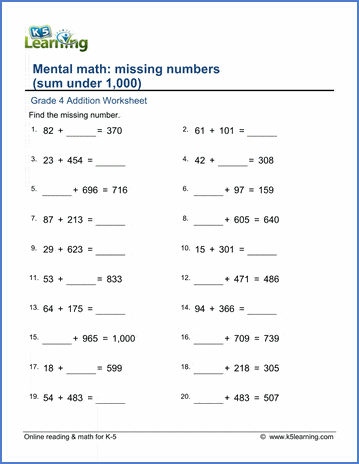# Addition with missing number (sum under 1,000)

## Math worksheets: 2 and 3 digit addition with missing numbers

Below are six versions of our grade 4 math worksheet on addition of 2 and 3 digit numbers; the student must find the missing numbers in each addition equation.  All sums are less than 1,000.  These worksheets are pdf files.